Function Repository Resource:

# FactorSemiprime

Fast integer factorization of a certain class of semiprimes

Contributed by: Sam Blake
 ResourceFunction["FactorSemiprime"][n] computes the prime factorisation of the semiprime, n.

## Details and Options

Given positive integers a,b such that a>b and a semiprime N, we may compute a factor, q, of N by creating the sequence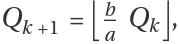where Q0=N. The sequence terminates if Qk<2, in which case the method has failed for the a/b-rational base representation. Otherwise, the sequence terminates if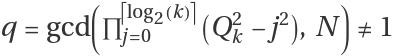, in which case we have found a factor, q, of N.
Similar to Fermat's factorisation algorithm, which factors N=p q quickly when p is close to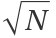, ResourceFunction["FactorSemiprime"] will factor N=p q quickly when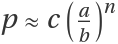, with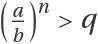and a,b are exhaustively searchable.
Similar to trial division, which tests successive odd integers for divisibility, ResourceFunction["FactorSemiprime"] exhaustively tests each possible a/b-rational base representation of p in lexicographic order. That is, a/b=2,3,4,3/2,5,6,5/2,4/3,7,5/3,
However, unlike trial division, for a single a/b, if, then ResourceFunction["FactorSemiprime"] will obtain the factor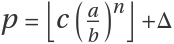, for all c∈ℕ and for all Δ∈ℕ such that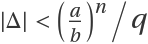.
Much like other existing algorithms for integer factorisation, it is exceedingly unlikely that ResourceFunction["FactorSemiprime"] will quickly factor an arbitrary, large semiprime.
ResourceFunction["FactorSemiprime"] cannot quickly factor any semiprimes from the RSA Factoring Challenge.

## Examples

### Basic Examples (1)

Factoring a small semiprime:

 In:=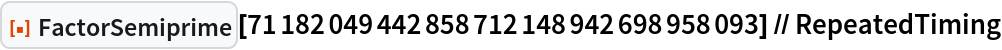Out=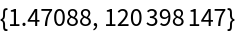### Scope (4)

Factoring a 1178-bit semiprime. This factorisation is faster than the previous example as the base representation of the larger prime factor is (exhaustively) searched sooner:

 In:=Out=Here we factor a semiprime, N=p q, where p is a prime close to (5/3)512 and p≫q:

 In:=Out=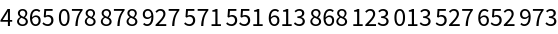In:=Out=Out=Out=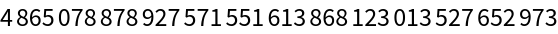Continuing this example, we can factor N=p q when p is close to 10001000100010003(5/3)555, where 10001000100010003 is an arbitrary integer coefficient:

 In:=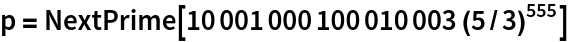Out=In:=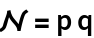Out=In:=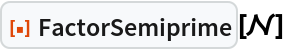Out=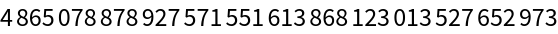We can still factor N=p q when p is Δ from 10001000100010003(5/3)555. That is p=10001000100010003(5/3)555±Δ, where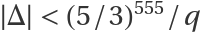:

 In:=Out=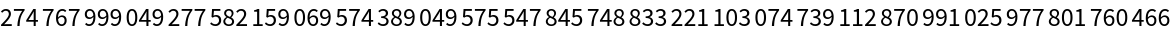Out=Out=Out=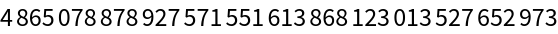In:=Out=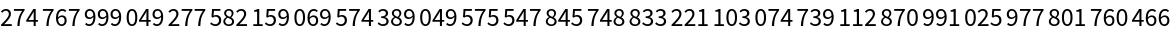Out=Out=Out=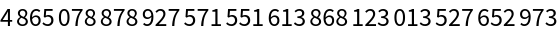### Options (1)

#### FactorSemiprimeMessages

 In:=#### InitialBase

 In:=Out=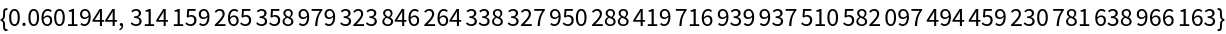#### SpecificBase (1)

Factoring RSA-250 by specifying the rational base. Unfortunately, we have no direct (non-exhaustive) way to compute or estimate this base without the factors:

 In:=Out=### Possible Issues (2)

Unlike FactorInteger, FactorSemiprime returns a single integer result:

 In:=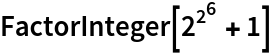Out=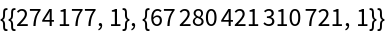In:=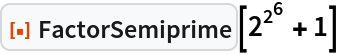Out=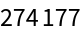If the input to FactorSemiprime is not a semiprime, then the product of one or more factors may be returned:

 In:=Out=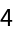In:=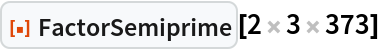Out=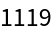### Neat Examples (1)

Factoring an enormous semiprime:

 In:=Out=Out=Out=Sam Blake

## Version History

• 1.0.0 – 12 September 2022

## Author Notes

At present we perform an exhaustive search on the a/b, it would be interesting to see if the continued fraction-type approach of Lehman could be adapted to this search.

The upper bound 1+Ceiling[Log[2,ndivs]] is heuristic, but seems to work well.